# Area of a Cylinder

Area of a Cylinder
Go back to  'Cylinder'

Have you seen a clown roll a drum standing on it?

The toilet paper roll, your can of cola? the pringles tin?

What do you think is common to all?

they all are cylindrical in shape.

Let us explore more about the cylinder area in this mini-lesson!

## Lesson Plan

 1 What is a Cylinder? 2 Area of a Cylinder 3 Important Notes 4 Interactive Questions 5 Challenging Questions 6 Frequently Asked Questions (FAQs)

## What is a Cylinder?

A cylinder is a three-dimensional shape with two parallel bases usually a circle and connected by a curved surface.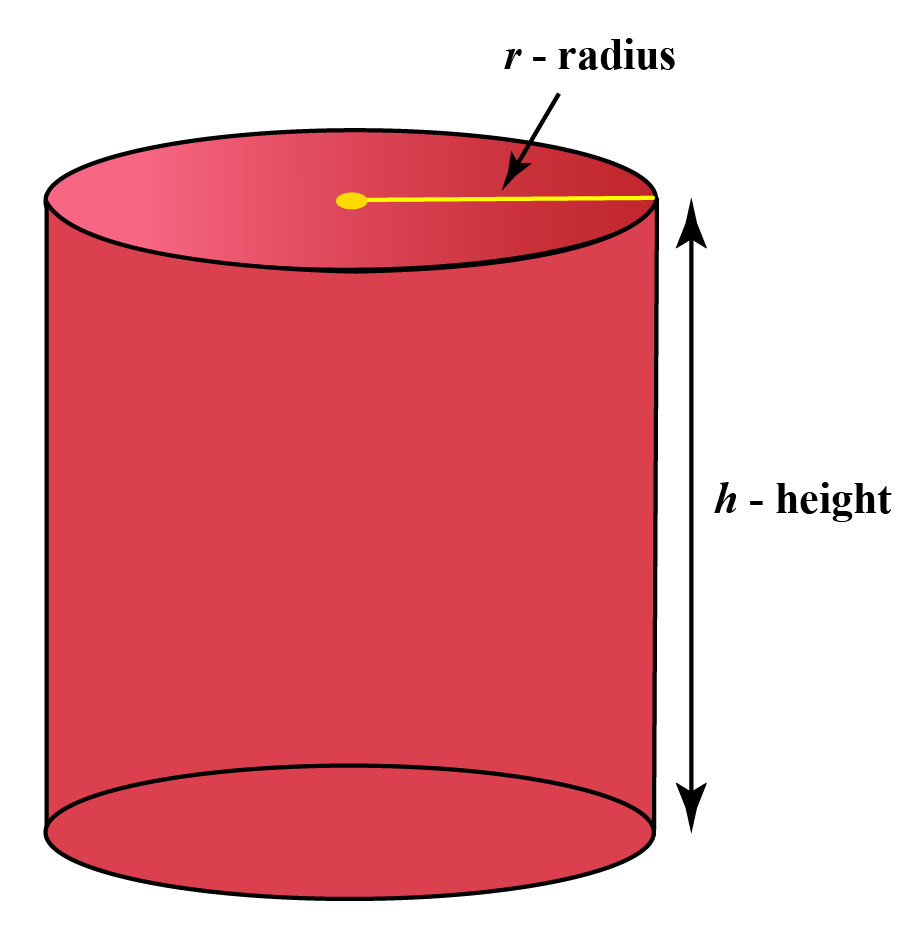Explore the cylinder here, drag the point r, h and visualize the cylinder.

## Area of a Cylinder

The area of any shape is the space occupied by it. A cylinder has 2 flat surfaces which are usually circle and a curved surface which opens up as a rectangle.

Consider the cylinder whose height is $$h$$  and a circular base with radius $$r$$.

Let us open a cylinder and see this.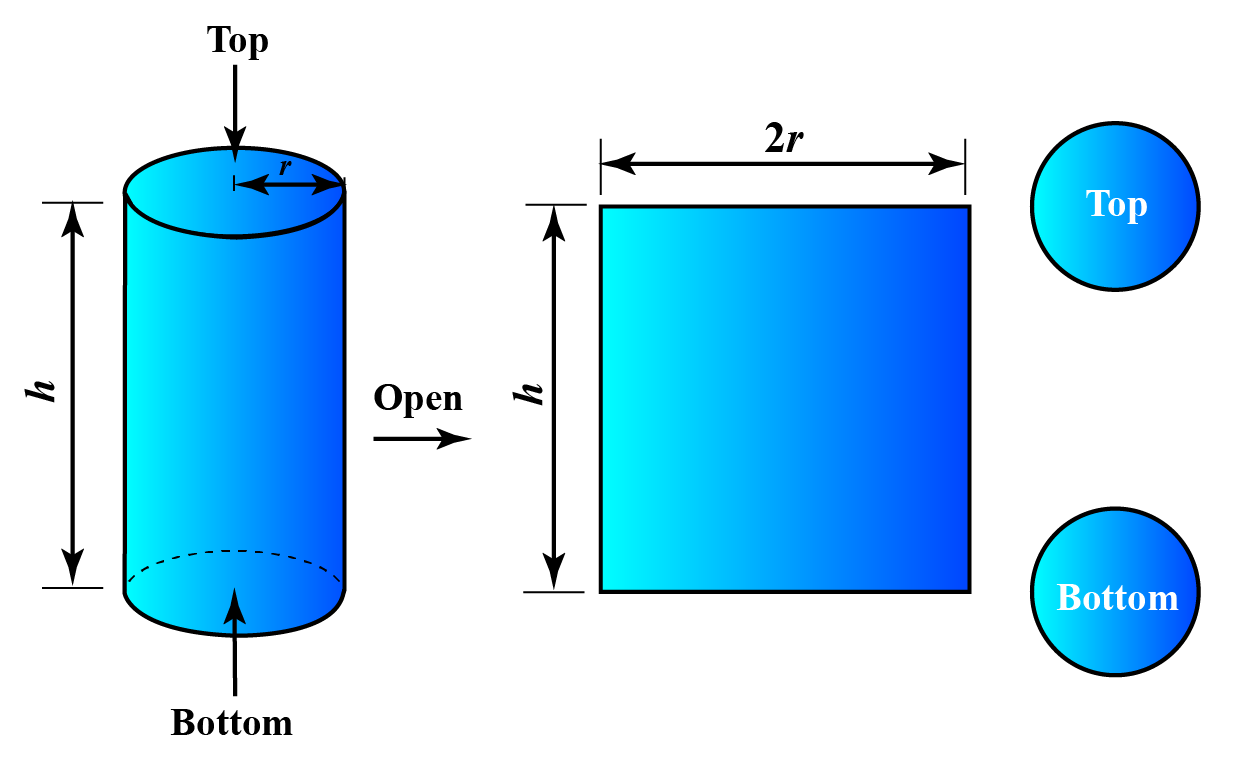So the area of the cylinder is the area of the two circles whose radius is 'r' and the area of the rectangle which is the curved surface.

The height of this rectangle is the height of the cylinder h, while the length of this rectangle is the circumference of the circle, that is, $$2\pi r$$. Thus, the area of this rectangle is the curved surface area of the cylinder, is $$2\pi rh$$.

Still confused,

look at the simulation below

Note that the volume of the cylinder is different from the area of the cylinder.

Volume is the quantity of space enclosed by the surface of the cylinder.

## Area of a Cylinder Formula

We just saw that a cylinder of height $$h$$ has 2 flat surfaces which are circles of radius $$r$$ and a curved surface that opens to a rectangle.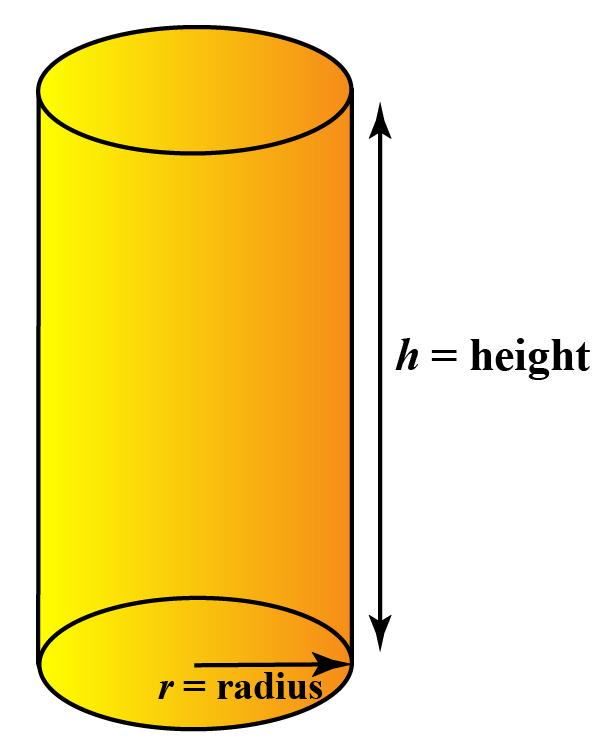The flat surface area of a cylinder is:

 Flat SA= $$2\pi {r^2}$$

The curved surface area of a cylinder is:

 Curved SA = $$2\pi rh$$

The lateral surface of a cylinder is the area of the cylinder, excluding the base which is:

 Lateral SA = $$2\pi rh$$

Total Surface Area is the area of flat surface and area of the curved surface which is:

 Total SA = $$2\pi {r^2} + 2\pi rh = 2\pi r\left( {r + h} \right)$$

The volume of the cylinder is the base area times the height, which is

 V = $$\pi r^2h$$

## Area of a Cylinder Examples

 Example 1

A cylinder has a radius of 3 in and a height of 10 in. Find its total surface area.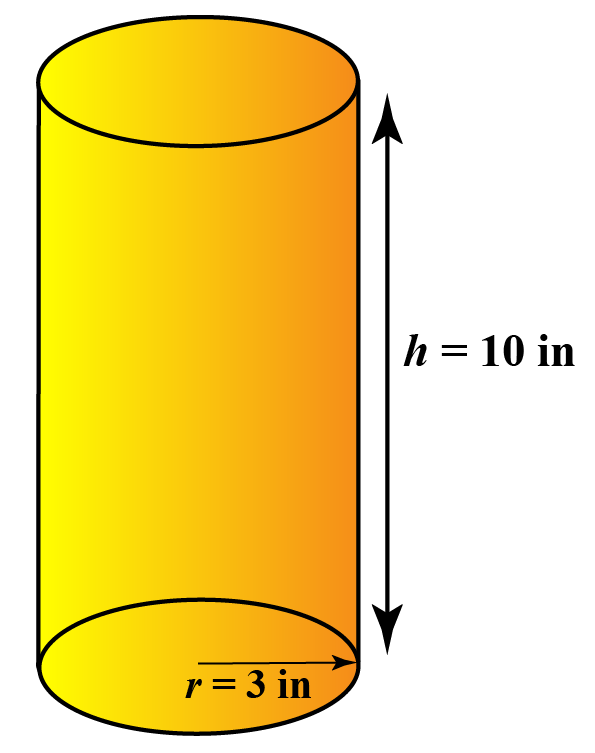Solution

\begin{align}{\rm{Total}}\,{\rm{SA}} &= 2\pi r\left( {r + h} \right) \\&= 78\pi \,{\rm{i}}{{\rm{n}}^2}\\ & \approx 245\,{\rm{i}}{{\rm{n}}^2}\end{align}

 $$\therefore$$ Total surface Area  = 245 sq.inches
 Example 2

Find the curved surface area of the given cylinder.

use $$\pi = 3.14$$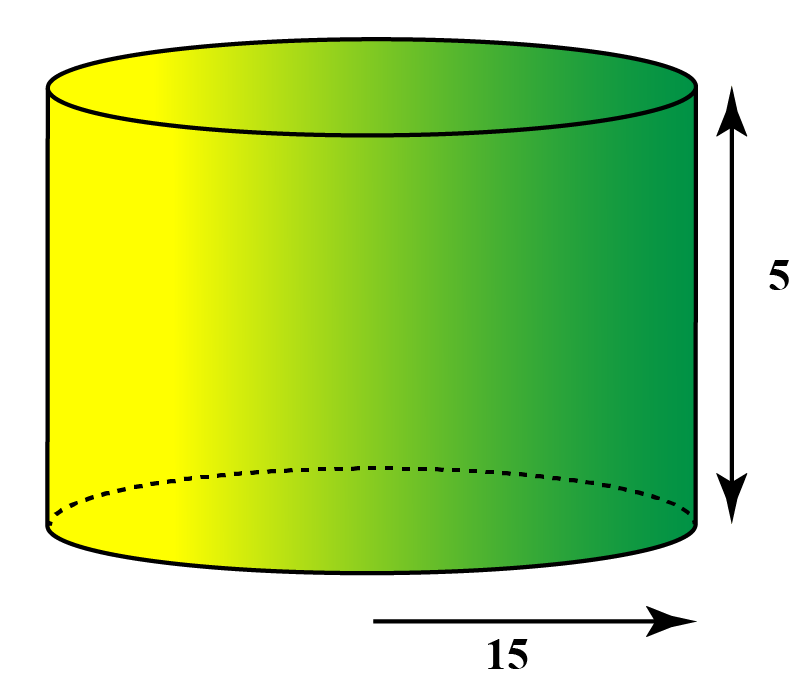Solution

The curved surface area of the cylinder = $$2\pi rh$$

Given r = 15  and h = 5

Substituting the values, we get

Curved surface Area = $$2 \times \dfrac{22}{7} \times 15 \times 5 \approx 471.42$$

 $$\therefore$$ Curved surface Area =   471.42  sq. units

## Area of a Cylinder Calculator

Enter the radius and height of the cylinder, the calculator gives the Flat surface area, curved surface area, and total surface area of the cylinder.

## Area of a Cylinder Problems  & Solutions

 Example 1

The total surface area of a cylinder is 880 sq. units. If the radius o the base is 7 units. Find the height of the cylinder.

USe $$\pi = dfrac{22}{7}$$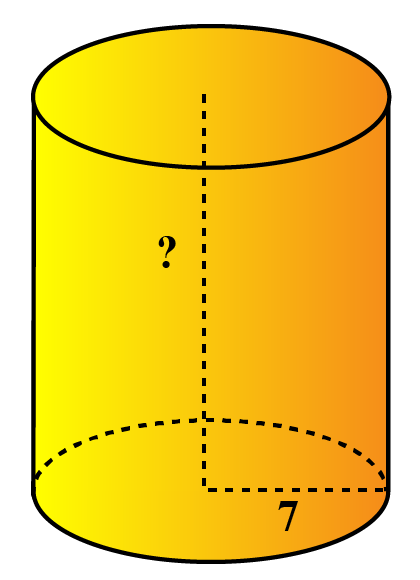Solution

Given

TSA = 880 sq units

We know that TSA = $$2\pi r\left( {r + h} \right)$$

Substituting the values,

we get
\begin{align} 880 &= 2 \times \frac{22}{7} \times 7 \left(7+ h \right)\\ 880 &=44 \left(7+ h \right) \\ \frac{880}{44} &= 7+h \\ 20 &= 7 +h \\ \implies h &= 20-7 \\ & = 13 \text{units} \end{align}

 $$\therefore$$  height of the given cylinder = 13 units
 Example 2

Rody wanted to paint the sides of the cylindrical vase. The base of the vase is a circle of radius of 3.5 units and the height of the vase is 7 units.

Find the area Rody has to paint.

use $$\pi = \frac{22}{7}$$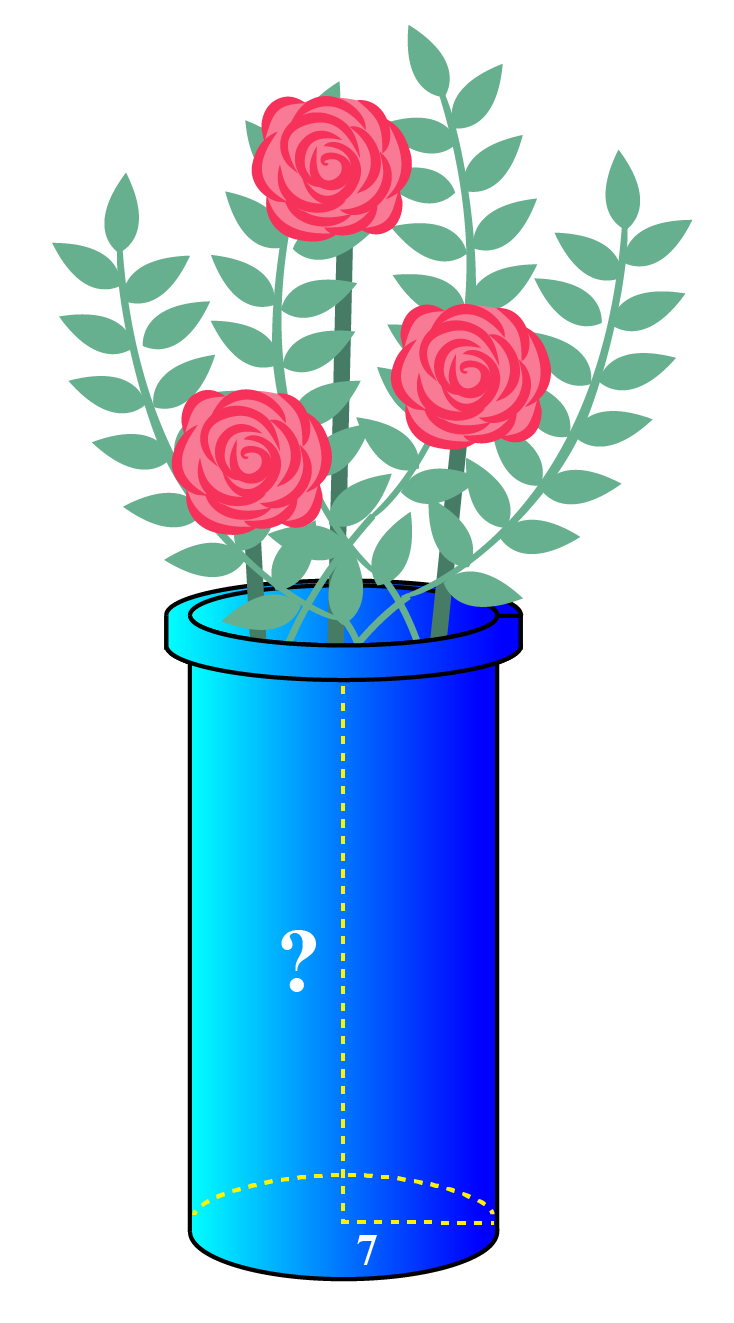Solution

Rody is painting the curved surface area of the vase.

The CSA of a cylinder is $$2\pi rh$$

substituting  r = 3.5 units and h = 7 units

we get

CSA = $$2 \times \frac{22}{7} \times 3.5 \times 7$$

CSA =  154 sq . units

 $$\therefore$$ Rody has to paint an area of  154 sq. units
 Example 3

The cost of painting a cylindrical container is $0.04 cm2. Jim has to paint 20 cylinders of height 50 cm and base radius of 20 cm. Find the total cost of painting. Use $$\pi = 3.14$$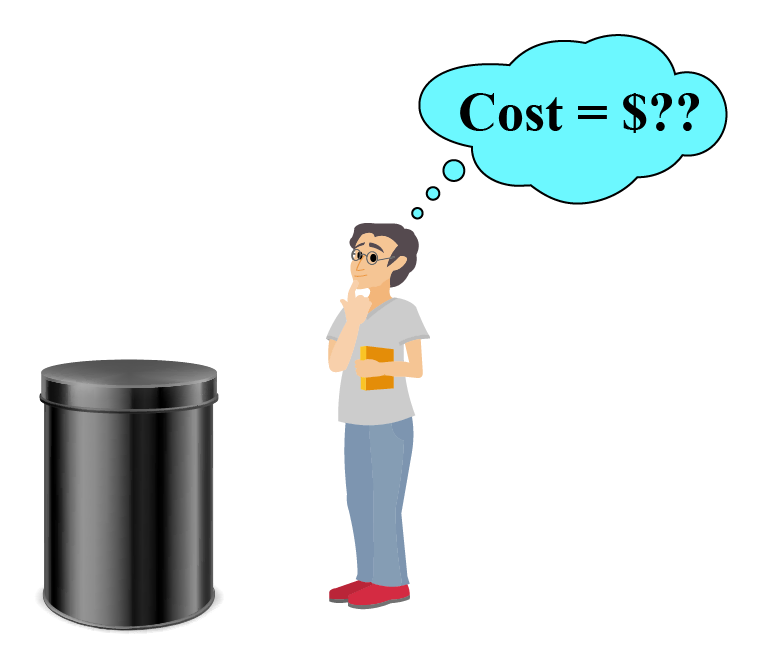Solution We need to find the cost of painting 20 cylinders total surface area We know that TSA = $$2\pi r\left( {r + h} \right)$$ TSA of 1 cylinder = $$2 \times 3.14 \times 20 \left( {20 + 50} \right)$$ Simplifying further we get TSA = 8792 cm2 Total surface area of 20 such cylinders = $$8792 \ times 20 = 175840 \text{cm}_2$$ Cost of painting 20 cylinders = $$175840 \times 0.04$$ =$7033.60

 $$\therefore$$ Cost of painting  20 cylinders = \$7033.60

## Interactive Questions

Here are a few activities for you to practice. Select/Type your answer and click the "Check Answer" button to see the result.Challenging Questions
1. Find the height of a cylinder whose volume is equal to the surface area of the cylinder.
2. The base radius and the length of the cylindrical roller of a  road roller are 0.8 m and 7 m respectively. What is the minimum number of revolutions required to flatten a road of width 7 m and length 4.4 km?

## 1. How do u find the area of a cylinder?

1. Flat Surface area of a cylinder = $$2\pi {r^2}$$  , where $$r$$ is the radius of the base circle
2. Curved Surface area of a cylinder  = $$2\pi rh$$ where $$h$$ is the height of the cylinder and $$r$$ is the base radius
3. Total Surface area of the cylinder of height $$h$$ and base radius $$r$$ = $$2\pi {r^2} + 2\pi rh = 2\pi r\left( {r + h} \right)$$

## 2. What is the area and volume of a cylinder?

Total Surface area of the cylinder of height $$h$$ and base radius $$r$$ = $$2\pi {r^2} + 2\pi rh = 2\pi r\left( {r + h} \right)$$ and volume is πr^2h.

## 3. What Is the Surface Area of a Cylinder?

The total surface area of the cylinder is the sum of the area of the top circle, bottom circle and the curved surface.

Total Surface area of the cylinder of height $$h$$ and base radius $$r$$ = $$2\pi {r^2} + 2\pi rh = 2\pi r\left( {r + h} \right)$$

More Important Topics
Numbers
Algebra
Geometry
Measurement
Money
Data
Trigonometry
Calculus